# Acid, bases and saltsPage 3

#### WATCH ALL SLIDES

Mg(s) + H2SO4(aq)  MgSO4(aq) + H2(g)

With bases (metal oxides and hydroxides)

The base dissolves in the acid and neutralises it. A salt is formed.

H2SO4(aq) + CuO(s)  CuSO4(aq) + H2O(l)

With metal carbonates

With metal carbonates, effervescence occurs, salt, water and carbon dioxide gas is produced.

2HCl(aq) + CaCO3(s)  CaCl2(s) + H2O(l) + CO2(g)

Slide 15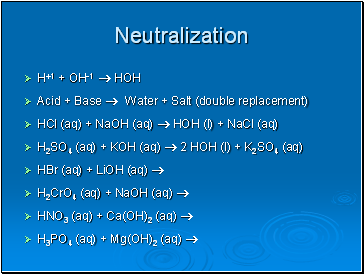## Neutralization

H+1 + OH-1  HOH

Acid + Base  Water + Salt (double replacement)

HCl (aq) + NaOH (aq)  HOH (l) + NaCl (aq)

H2SO4 (aq) + KOH (aq)  2 HOH (l) + K2SO4 (aq)

HBr (aq) + LiOH (aq) 

H2CrO4 (aq) + NaOH (aq) 

HNO3 (aq) + Ca(OH)2 (aq) 

H3PO4 (aq) + Mg(OH)2 (aq) 

Slide 16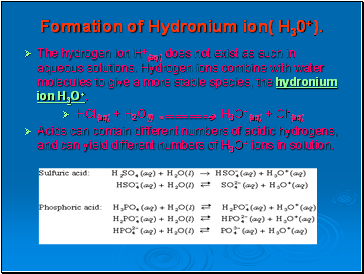## Formation of Hydronium ion( H30+).

The hydrogen ion H+(aq) does not exist as such in aqueous solutions. Hydrogen ions combine with water molecules to give a more stable species, the hydronium ion H3O+.

HCl(aq) + H2O(l) < ========= H3O+(aq) + Cl-(aq)

Acids can contain different numbers of acidic hydrogens, and can yield different numbers of H3O+ ions in solution.

Slide 17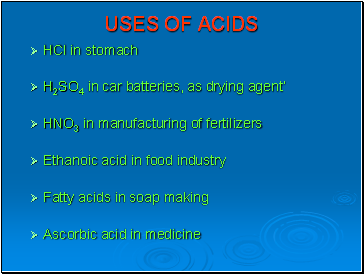## Uses of Acids

HCl in stomach

H2SO4 in car batteries, as drying agent’

HNO3 in manufacturing of fertilizers

Ethanoic acid in food industry

Fatty acids in soap making

Ascorbic acid in medicine

Slide 18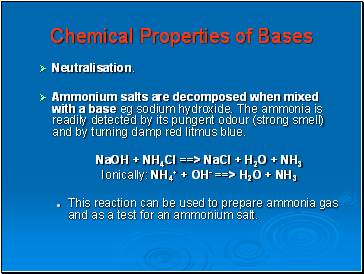## Chemical Properties of Bases

Neutralisation.

Ammonium salts are decomposed when mixed with a base eg sodium hydroxide. The ammonia is readily detected by its pungent odour (strong smell) and by turning damp red litmus blue.

NaOH + NH4Cl ==> NaCl + H2O + NH3

Ionically: NH4+ + OH- ==> H2O + NH3

This reaction can be used to prepare ammonia gas and as a test for an ammonium salt.

Slide 19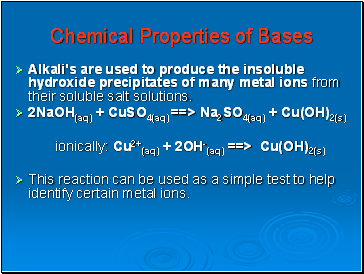## Chemical Properties of Bases

Alkali's are used to produce the insoluble hydroxide precipitates of many metal ions from their soluble salt solutions.

2NaOH(aq) + CuSO4(aq) ==> Na2SO4(aq) + Cu(OH)2(s)

ionically: Cu2+(aq) + 2OH-(aq) ==> Cu(OH)2(s)

This reaction can be used as a simple test to help identify certain metal ions.

Slide 20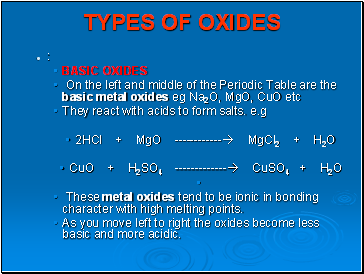## Types of Oxides

:

BASIC OXIDES

On the left and middle of the Periodic Table are the basic metal oxides eg Na2O, MgO, CuO etc

They react with acids to form salts. e.g

2HCl + MgO ------------ MgCl2 + H2O

Go to page:
1  2  3  4  5  6  7